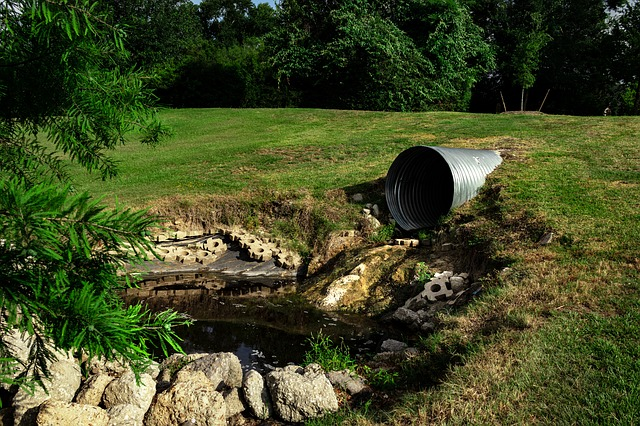Feb 26, 2016

Feb 26, 2016

Feb 26, 2016

# How to Design Sanitary Sewer using Manning's Equation## Sanitary Sewer Design step wise procedure:

### Step 1: Finding the Design Flow

1. Calculate the anticipated future (ultimate) population of area served by the sewer using ultimate population density.
2. Multiply the ultimate population by per capita average daily sewage flow to obtain the average daily sewage flow e.g.
3. Select or calculate peaking factor (P.F) & Peak hourly waste water flow

P.F = or

Or

P.F = with max of 4

P.F = 4

Where P = Population in 1000 & Q = Flow in MGD

Now

Peak hourly domestic flow = P.F x Qw

4. Calculate the infiltration allowance at the rate of 10% of average daily waste water flow

or (3% to 5% of peak hourly domestic flow)

or 200 to 500 gallons/mile/inch-dia

or 700 to 1500 gallons/day/acre

or 30,000 (gallons per day) infiltration per mile of sewer

or 170,000 (liters per day) infiltration per km & house connections.

5. Calculate the allowance for industrial and commercial sewage at the rate of 3,734 m3/km2/day or 4,000 gal/acre/day.

Design flow for sanitary sewer =

Peak hourly domestic + Infiltration + Commercial waste water flows

### Step 2: Finding velocity using Manning formula

(m/s, m, mm)

(ft/s, ft, ft/ft)

### Step 3: Check for minimum self cleaning velocity

V > Vmin (Vmin = 0.60 m/sec OR 2 ft/sec)

### Step 4: Finding the Diameter of Sewer pipe

Design Flow = X-sec Area of pipe * Velocity

Q = A*V

Where A =

From the above you can find the diameter of the pipe